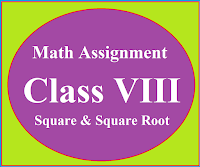### Math Assignment Class VIII | Square & Square RootMath Assignment  Class VIII | Square & Square Root Download or Print free  assignment with answer key  for   Class  8 Squares and  Square Roots.   Important and extra questions that cover all topics of square and square root and is useful and helpful for the students. Math Assignment  Class VIII | Square & Square Root LEVEL -1

### Class IX Maths Formulas | Basics | Assignments

CBSE Class IX Maths Syllabus and Sample Papers
Study Material for Class VIII (8th)
CBSE syllabus, sample papers, unit tests and solutions of sample papers, CBSE syllabus for class IX and VIII for all subjects, chapter-wise basic concepts of mathematics for class IX, chapter-wise planning in mathematics for class IX, Study material for class VIII.

# CHAPTER WISE EXPLANATION

## CHAPTER 1:  Number System (Algebra)

Basics & Formulas

Assignment

Multiple Choice Questions Ch-1 Class IX

Solved Explanation of the questions

Lesson Plan Mathematics

## CHAPTER 2:  Polynomials

Basics & Formulas

Assignment

Multiple Choice Questions Ch-2 Class IX

Algebraic Identities

Lesson Plan Mathematics

## CHAPTER 3:  Coordinate Geometry

Basics & Formulas

Assignment

Lesson Plan Mathematics

## CHAPTER 4: Linear Equations in two variables

Multiple Choice Questions Ch-4 Class IX

Lesson Plan Mathematics

## CHAPTER 5: Euclid's Geometry

Lesson Plan Mathematics

## CHAPTER 6: Lines and angles (Geometry)

Basics & Formulas

Multiple Choice Questions Ch-6 Class IX

Lesson Plan Mathematics

## CHAPTER 7: Triangles (Geometry)

Congruence of Triangles

Properties of Triangle

Lesson Plan Mathematics

Basics & Formulas

Theorems on chapter 8

Multiple Choice Questions Ch-8 Class IX

Lesson Plan Mathematics

## CHAPTER 9: Area Related to Quadrilaterals

Basics & Formulas

Planning

## CHAPTER 10: Circles (Geometry)

Basics & Formulas

Multiple Choice Questions Ch-10 Circle

Theorems on Circle Ch-10

## CHAPTER 11: Constructions (Geometry)

This Chapter is deleted for the session (2022-23)

Planning

## CHAPTER 13:  Surface area and Volumes (Solid Geometry)

Basics & Formulas

Multiple Choice Questions Ch-13 Surface Area & Volume

Planning

## CHAPTER 14:  Statistics (Mean, Mode, Median)

Basics & Formulas

Mathematics MCQ Class IX Ch-14 | Statistics

Planning

## CHAPTER 15: Probability (Tossing of Coins & Dice)

Deleted for session 2022-23

Basics & Formulas

Assignments

Multiple Choice Questions Ch-15 Probability

 NCERT Mathematics Book with solutions NCERT Book NCERT Sol. NCERT Book NCERT Sol.

 STUDY MATERIAL FOR CLASS VIII (8TH)DAV BOARD (All Subjects) Social Science Previous Question Papers and other study material Science Previous Question Papers and other study material Mathematics Previous Question Papers and other study material English Previous Question Papers Bank Hindi Previous Question Papers Bank

DAV Sample Paper For Class VIII
 DAV – SP-1 –Maths - 2020-21 Sol-1- (Maths) DAV – SP-2 –Maths - 2020-21 Sol-2- (Maths)

For more blogs please visit the site